# Thread: dB/Hz Frequency spectrum out of fftw

1. ##dB/Hz Frequency spectrum out of fftw

I am trying to get spectrum data (the numbers, not the graphics) out of FFTW, like this (from Audacity):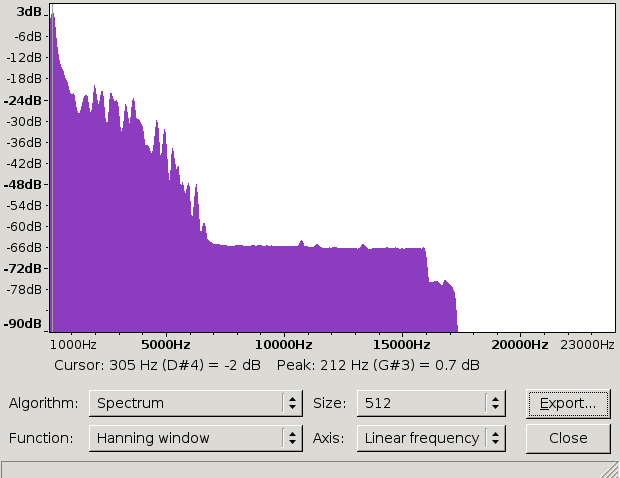After studying fftw's documentation and all Google links I could find I am still unable to interpret the output of FFTW correctly. I just don't know where to find which frequency in there.

Here is what I got. It is obviously center-plotted but let's leave that aside, it doesn't look any good if you shift it.

Linear X scale: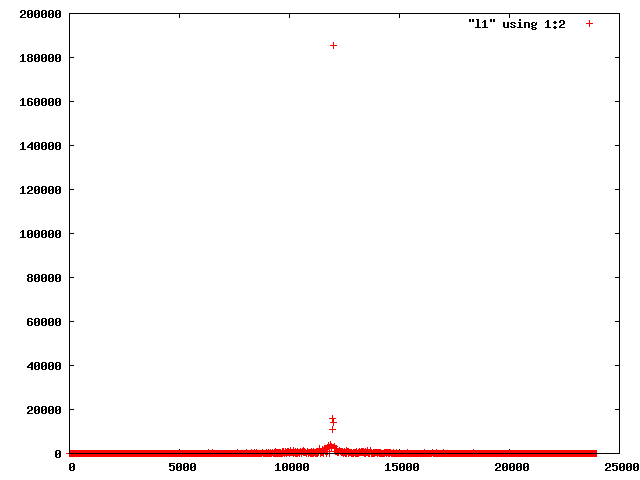Logarithmic: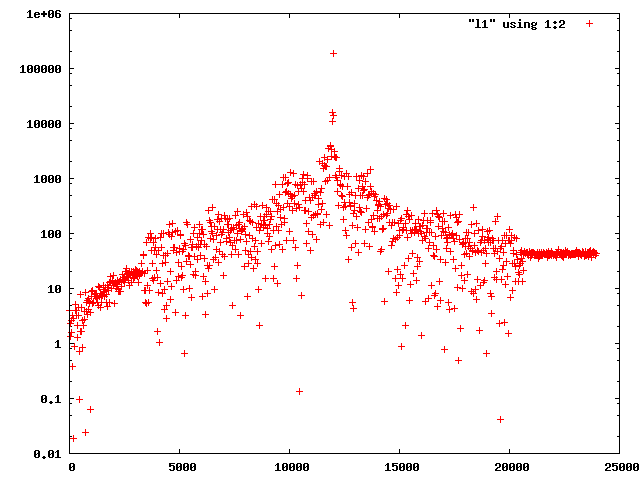Any idea what I am doing wrong here? The code is a routine from a larger program, I can make a runnable thing available if you like.

Code:
```struct frames {
int n_frames;
int n_files;
char **files;
frames(): n_frames(-1), n_files(-1){}
};

typedef double fftw_input_type;
typedef double fftw_output_type;
typedef double fftw_spectrum_type;

static int fft(const struct frames &frames)
{
int n_points = frames.n_frames;

n_points = 50000; // short sample

fprintf(stderr, "In fft on %d samples\n", n_points);

fftw_input_type *in;
fftw_output_type *out;
fftw_complex *comout;
fftw_plan plan;
fftw_plan cplan;

fftw_spectrum_type *spectrum;

in = (fftw_input_type *) fftw_malloc(sizeof(fftw_input_type) * n_points);
out = (fftw_output_type *) fftw_malloc(sizeof(fftw_output_type) * n_points);
comout = (fftw_complex *) fftw_malloc(sizeof(*comout) * n_points);
spectrum = (fftw_spectrum_type *)malloc(sizeof(*spectrum) * n_points / 2 + 1);

plan = fftw_plan_r2r_1d(n_points, in, out, FFTW_R2HC, FFTW_FORWARD);
cplan = fftw_plan_dft_r2c_1d(n_points, in, comout, FFTW_FORWARD);

channeldata *data1 = (channeldata*)frames.files;

for (int i = 0; i < n_points; i++) {
// fixme, channel 0 only
in[i] = (double)data1[i].channels;
}

fftw_execute(plan); /* repeat as needed */
fftw_execute(cplan); /* repeat as needed */

spectrum = out * out;
int n_spectrum_points = (n_points + 1) / 2;
for (int k = 1; k < n_spectrum_points; k++)  /* (k < N/2 rounded up) */
spectrum[k] = out[k] * out[k] + out[n_points - k] * out[n_points - k];
if (n_points % 2 == 0) /* N is even */
spectrum[n_points / 2] = out[n_points / 2] * out[n_points / 2];  /* Nyquist freq. */

#if 0
for (int i = 0; i < n_spectrum_points; i++) {
printf("Spectrum: %f\n", spectrum[i]);
}
#endif

double correction = (double)sample_frequency / (double)n_points / 2;
double accu = 0;
double freq1 = -1;
double freq2 = -1;
const int every_nth = n_points / 1000; // want this number of lines
// rotate output array by half
int max = n_points - 2;
for (int k = 0; k < max; k++) {
int index_in_output = k + max / 2;
if (index_in_output > max)
index_in_output -= max;

double absval = sqrt(out[index_in_output] * out[index_in_output]);
double cc = (double)k * correction;
double maybe_freq = (double)k / (double)n_points;
if (k % every_nth == 0) {
if (k != 0) {
freq2 = cc;
// FIXME - last value documented to be lost
printf("%f %f %f %f ( %f - %f ) %f\n"
, (freq2 + freq1) / 2.0
, absval / every_nth
, sqrt(comout[index_in_output] * comout[index_in_output])
, sqrt(comout[index_in_output] * comout[index_in_output])
, freq1, freq2
, maybe_freq
);
}
freq1 = cc;
accu = 0;
}
accu += absval;
}

fftw_destroy_plan(plan);
fftw_free(in);
fftw_free(out);

return 0; // success, this goes to exit
}```Adv Reply

2.Frothy Coffee!Join Date
Jul 2006
Beans
209

## Re: dB/Hz Frequency spectrum out of fftw

Hello uOpt,

the fact you're asking how to find a given freq in an FFT makes me think you're not familiar with the discrete fourier transform (DFT).... bear with me a small explanation...

The main idea is that you have N (in principle complex) samples corresponding to a time duration of T, let's call those samples S_n, with n going from 0 to N-1. The result of applying the DFT to that vector is another complex vector of N elements, let's call it F_n. As you are expecting, F_n is related to a frequency component of the original vector. In fact, F_n corresponds to the fourier component of frequency w_n = 2*pi*n/T. Because of aliasing (ignore the term if you want) things are a bit more complicated. You can use the formula above for n up to N/2. For larger n, F_n corresponds to negative frequencies: w_n=-2*pi*(N-n)/T.
Now, for a given frequency, there are two quantities, magnitude and phase. You're interested in the magnitude, which you can get by taking the absolute value squared of the fourier component corresponding to the given frequency plus the absolute value squared of the fourier component corresponding the the negative of the given frequency.
I hope you're still with me. Let's recap for a second. DFT gives you frequency information for frequencies ranging from -pi*N/T to pi*N/T in discrete intervals. The smallest frequency you can get is 2*pi/T, which makes sense, since it correspond to a period of T which is all you have in you initial vector. The highes freq you have is pi*N/T which correspond to a period of T/(2N). Again it makes sense, because you cannot know what happens in between the input samples.

Now FFT is a fast method of calculating DFT, and FFTW can do that. However, one has to be careful about the format of the input and output vectors. FFTW tries to take advantage of the fact that if the input array is real (not complex), then there is some redundancy in the output vector. In fact, if the input array is real (as in your case), the fourier component of a frequency w is equal to the complex conjugate of the fourier component of the freq -w. So half the vector can be known from the other half. That means that in fact, we only need (N/2)+1 complex numbers for the output (as opposed to N complex numbers).
There are different ways of storing those numbers in the output array, depending on how you call FFTW. If you use the r2c plan, it will just be an vector of N/2+1 fftw_complex numbers (f_i) with freqs corresponding to 2*pi*i/T.
If you use the r2r plan, it is more complicated. The output is an array of N real numbers, like this:
r0, r1, r2, ...., r_(n/2), i_(n+1)/2-1, ...., i2, i1
where (r0,0) is f_0, (r1,i1) is f_1, and so on (f_0 does not have imaginary part because the input array is real).

It is not necessary to call both plans as you do in your code, you're computing the same thing twice. You can just call one of the plans (the one you like better) and do all your calculations based on that.

The line
spectrum = (fftw_spectrum_type *)malloc(sizeof(*spectrum) * n_points / 2 + 1);
has a bug, it's missing a parenthesis. It should be
spectrum = (fftw_spectrum_type *)malloc(sizeof(*spectrum) * (n_points / 2 + 1));

Well I hope I made some sense.

EDIT: I just realized that what I call frequency is not actually frequency, it is some sort of "wave number". When I say the frequency is 2*pi/T, for instance, I mean a "real" frequency of 1/T. So whenever I say frequency, divide by 2pi.
Last edited by croto; May 22nd, 2010 at 08:20 PM.Adv Reply

3.Just Give Me the Beans!Join Date
Nov 2009
Location
/dev/null
Beans
74

## Re: dB/Hz Frequency spectrum out of fftw

I didn't look into the details of your program, but I noticed n_points=50000.
Not sure what algorithm you're using, but if you're using Radix-2(which is popular), the number of point must be a power of 2 or have to be adjusted accordingly.
Just what came up in my mind...
Last edited by lostinxlation; May 23rd, 2010 at 06:30 AM.Adv Reply

4. ## Re: dB/Hz Frequency spectrum out of fftw

You might look into GSL ( GNU Scienific Library ) for this. I use it for calculating power spectral density. You should use a data window on your data first. Like Nutall or Blackman, etc. It's like a filter. Then perform the FFT. You might try averaging. After that you need to scale the FFT ouput to dB. If the input to the FFT is in units of Volts then the output will be Volts^2 per line. In you plots you are plotting both halves of the FFT output. That's why it looks different than the Audicty plot.Adv Reply

5. ## Re: dB/Hz Frequency spectrum out of fftw

nm
Last edited by Cracauer; May 24th, 2010 at 06:48 PM.Adv Reply

6. ## Re: dB/Hz Frequency spectrum out of fftw

It's beginning to make more sense now, but one potentially stupid question. I'm trying to get the input right first.

I can feed in an audio clip with as many full waves as I want, right?

So if I'm supposed to pick a power of two number of input samples, then I will definitely have the end of the clip at an amplitude of non-zero. It is not clear to me how I am supposed to put "made-up" sample around my actual samples that make the clip begin and end at amplitude zero. Wouldn't that change the spectrum?

I am now experimenting with feeding in just a 440 Hz sine wave, that should allow me to see when things begin to make fall in and how to convert to frequency later.

I deliberately computed two variants although I know I only need one, I just thought I look at either to see if the result make more sense one (didn't of course.
Last edited by uOpt; May 24th, 2010 at 11:49 PM.Adv Reply

7. ## Re: dB/Hz Frequency spectrum out of fftw

I use a value of 131072 samples in my PSD function. That's 128K. I use a samples rate of 8000 samples/sec. (telephony) It doesn't matter whether the wave form starts or stops at the zero-crossing.

The tricky part is finding out how the fft routine outputs the results. Most of the time it's 'in-place' and you have to move something around.
For instance this is what I do using GSL:
Code:
```(void) gsl_fft_real_radix2_transform(v, 1, (size_t) len);

v = SQR(v) * psdscale;
double vnyquist = SQR(v[len / 2]) * psdscale;

for (int k = 1; k < len / 2; k++)
v[k] = 2.0 * (SQR(v[len - k]) + SQR(v[k])) * psdscale;

v[len / 2] = vnyquist;```Adv Reply

8.Frothy Coffee!Join Date
Jul 2006
Beans
209

## Re: dB/Hz Frequency spectrum out of fftwOriginally Posted by uOptIt's beginning to make more sense now, but one potentially stupid question. I'm trying to get the input right first.

I can feed in an audio clip with as many full waves as I want, right?
If I understand correctly, you're asking if the input can have as many full "oscillations" as you want? I guess you're thinking of a pure sine wave as you mentioned. The answer is yes. In fact, the more full waves you have, the better the fourier analysis will be able to resolve that frequency.Originally Posted by uOptSo if I'm supposed to pick a power of two number of input samples, then I will definitely have the end of the clip at an amplitude of non-zero. It is not clear to me how I am supposed to put "made-up" sample around my actual samples that make the clip begin and end at amplitude zero. Wouldn't that change the spectrum?
For FFTW the input does not need to be a power of two. It is true that if it is a power of two it works faster (if I remember correctly, the algorithm works fastest if the length of the input can be factored as 2^i * 3^j * 5^k (something like that, check FFTW documentation)).
It does not matter if the clip end of starts at amplitude zero. The spectrum should be invariant under (cyclical) shift of the input.Originally Posted by uOptI am now experimenting feeding in just a 440 Hz sine wave, that should allow me to see when things begin to make fall in and how to convert to frequency later.
Good idea!Adv Reply

9. ## Re: dB/Hz Frequency spectrum out of fftw

Thanks so much, guys.

Here's a cleaned up version that gives me this for the 440 Hz sine wave: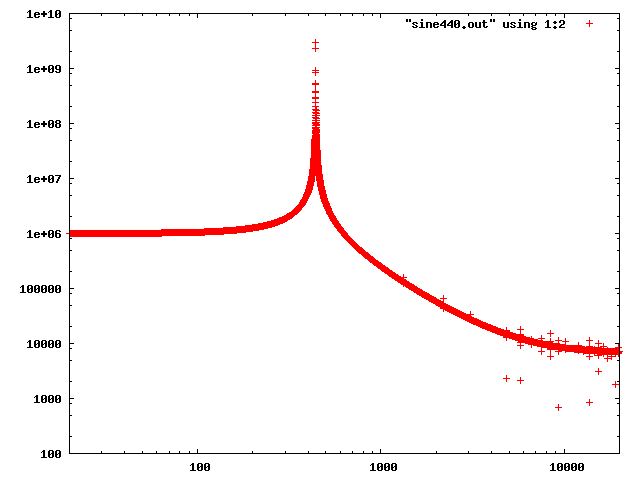But the spectrum on the audio clip (that should be the same as the audacity shot) is still a mess: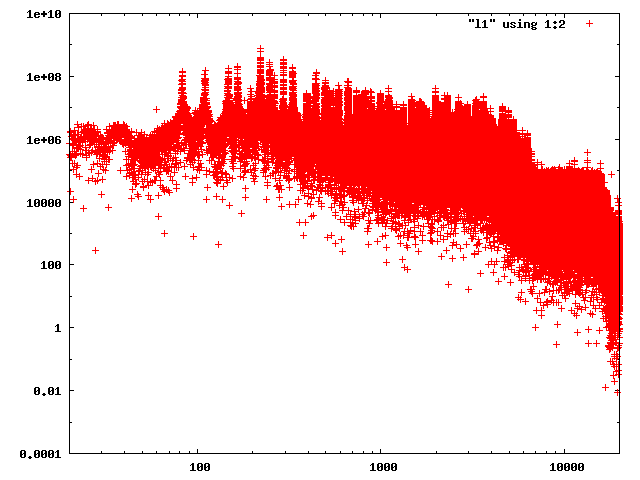Code:
```static int fft(const struct frames &frames)
{
int n_points = frames.n_frames;

fprintf(stderr, "In fft on %d samples\n", n_points);

fftw_input_type *in;
fftw_output_type *out;
fftw_complex *comout;
fftw_plan plan;

in = (fftw_input_type *) fftw_malloc(sizeof(fftw_input_type) * n_points);
out = (fftw_output_type *) fftw_malloc(sizeof(fftw_output_type) * n_points);
comout = (fftw_complex *) fftw_malloc(sizeof(*comout) * n_points);

plan = fftw_plan_r2r_1d(n_points, in, out, FFTW_R2HC, FFTW_FORWARD);

channeldata *data1 = (channeldata*)frames.files;
memset((void *)out, 0, sizeof(fftw_output_type) * n_points);
for (int i = 0; i < n_points; i++) {
// fixme, channel 0 only
in[i] = (double)data1[i].channels;
}

fftw_execute(plan);

{
int m = 0;
double correction = (double)sample_frequency / (double)n_points;
for (int i = 0; i < (n_points / 2) + 1; i++) {
double absval = sqrt(out[i] * out[i]);
double cc = (double)m * correction;
printf("%f %f\n", cc, absval);
m++;
}
}

fftw_destroy_plan(plan);
fftw_free(in);
fftw_free(out);

return 0;
}```Adv Reply

10.Frothy Coffee!Join Date
Jul 2006
Beans
209

## Re: dB/Hz Frequency spectrum out of fftw

It's looking better! I found a little bug, you're using the r2r plan, which puts the imaginary parts of the fourier components at the end of the output array. So you'd need to change the line
Code:
`double absval = sqrt(out[i] * out[i]);`
to
Code:
`double absval = out[i] * out[i] + out[(n_points / 2) + 1 - i] * out[(n_points / 2) + 1 - i];`
Also, the power is defined as the square, so you don't need the squared root.

I guess that change will make the spectrum look a bit cleaner. For extra cleanness, you'd have to use some filter. You're just cutting the signal (or using a square window)... another poster suggested using a weighted kind of window instead of the square one. That may help to make the spectrum look cleaner.

Also, for comparison with Audacity, it looks they use linear scale for the frequency axis.

EDIT: I didn't test the change in teh code I suggested... I just wanted to add the square of the corresponding imaginary part (you were squaring only the real part)Adv Reply

####Posting Permissions

• You may not post new threads
• You may not post replies
• You may not post attachments
• You may not edit your posts
•